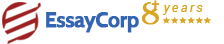WhatsApp
Struggling With Assignments?

We are here to help.

Chat with our expert writers on WhatsApp

for any kind of assignment queries.

Click to chat!
Struggling with Assignments? WhatsApp Us#### Q1. Ans.

If, before multiplication with a carrier, there is addition of a DC component to the message signal, then the modulation scheme is referred as amplitude modulation (AM). The objective of the DC component is to allow the modulated carrier to be demodulated at the receiver with the help of a means other than synchronous detection. For output that is envelope detector, the coupling should be set for AC. (So, there is completion of the envelope detection , there is no need of DC component.)

#### Q2. Ans.

It is significant that a key characteristic of an AM signal is seen by you- shape of its envelopes are the same as the message ( But there is inversion of the lower envelop).

#### Q3. Ans.

Within sample, there is presence of three type of sine waves. The two sine waves have a frequency of 100khz, 49 khz and 51 khz.#### Q4. Ans.

The multiplier module has been provided with a sine wave message signal and this sine wave signal appears at the message module.

#### Q5. Ans.

200mV of signal modulate A 1V amplitude carrier. It‘s amplitude varies from .8V to 1.2V. In case of there is an increase of signal to 300mV, there will be variation of amplitude from 0.7V to 1.3V. In case, there is an increase in carrier amplitude up to 2V, then variation in amplitude will be from 2.3 to 1.7 0.6 is the difference between valley of 1.7 and peak of 2.3. 2 is the average. Hence, modulation depth is (1/2) of difference between valley and peak divided by average *100%. Here, it is (0.3/2}*100% = 15%. 100% is the maximum permissible. In amplitude modulation the carrier wave amplitude is varied is varied linearly with the modulating wave (the base band signal) creating what is called the envelope signal. Shape of the envelope is same as the base band signal if: 1. The highest frequency components fm of the message signal m (t) must be less then the carrier frequency fc i.e. fc >> fm 2. Unity should be more then the modulation index. If it is other wise, then there is over modulation of the carrier Once we don't have the envelope, or the envelope doesn't resemble the original baseband signal, the detection of the AM signal to restore the modulating wave m(t) can't be done with a simple envelope detector (a diode followed by a low pass filter- RC circuit). Detection may be done, however, using other AM types of the suppressed carrier types.

#### Q6. Ans.

From the envelope itself, the modulation index can be estimated which will be shown on the oscilloscope. As there is an increase in the amplitude of the message signal, in the envelop there is an appearance of extra lobes. Over modulation is indicated by these lobes. As there is decrease of the amplitude of the message signal that is below 100% modulation, measurements of the minimum and the maximum envelope amplitude provides the modulation index. This modulation index is estimated with the help of this difference: m = 2/ 10[(carrier dB - sideband dB)/20]

The problem with am over modulation is that it causes distortion from the receiver point of view. For the transmitter, the problem is that some of the energy is now outside the frequency band in the output, and may even affect and hamper with other stations or other services.

#### Q7. Ans.

Modulation index is the peak change in the RF amplitude from its unmodulated value. For an AM modulation index of 0.5, the modulation causes the signal to multiply by a factor of 0.5 and even decrease to 0.5 of its original level.

Amplitude modulation depth

A complementary figure to modulation index is also used for amplitude modulation signals. Known as the modulation depth, it is typically the modulation index expressed as a percentage. Thus a modulation index of 0.5 would be expressed as a modulation depth of 50%, etc.

However often the two terms and figures are used interchangeably and figures for a modulation index of 50% are often seen where the index is 0.5.

#### Q8. Ans

Although the message and the carrier are periodic waveforms (sinusoids), the DSBSC itself need not necessarily be periodic.

#### Q9. Ans.

The upper side band , lower sideband and carrier frequency are present in the DSB am modulation.

#### Q10. Ans.

Considering the DSB-SC modulation, unlike in AM, the wave carrier is not a transmitted one; therefore, much of the power is distributed between the sidebands. This implies an increase of the cover in DSB-SC, compared to AM, for the same power used in the message.

HIRE EXPERT
Email ID *
Subject
Pages